NEET  >  NEET Previous Year Questions: Gravitation - 1

# NEET Previous Year Questions: Gravitation - 1

Test Description

## 22 Questions MCQ Test Physics Class 11 | NEET Previous Year Questions: Gravitation - 1

NEET Previous Year Questions: Gravitation - 1 for NEET 2023 is part of Physics Class 11 preparation. The NEET Previous Year Questions: Gravitation - 1 questions and answers have been prepared according to the NEET exam syllabus.The NEET Previous Year Questions: Gravitation - 1 MCQs are made for NEET 2023 Exam. Find important definitions, questions, notes, meanings, examples, exercises, MCQs and online tests for NEET Previous Year Questions: Gravitation - 1 below.
Solutions of NEET Previous Year Questions: Gravitation - 1 questions in English are available as part of our Physics Class 11 for NEET & NEET Previous Year Questions: Gravitation - 1 solutions in Hindi for Physics Class 11 course. Download more important topics, notes, lectures and mock test series for NEET Exam by signing up for free. Attempt NEET Previous Year Questions: Gravitation - 1 | 22 questions in 22 minutes | Mock test for NEET preparation | Free important questions MCQ to study Physics Class 11 for NEET Exam | Download free PDF with solutions
 1 Crore+ students have signed up on EduRev. Have you?
NEET Previous Year Questions: Gravitation - 1 - Question 1

### If suddenly the gravitational force of attraction between earth and a satellite revolving around it becomes zero, then the satellite will   [AIEEE 2002]

Detailed Solution for NEET Previous Year Questions: Gravitation - 1 - Question 1

When the gravitational force becomes zero that means the centripetal force required cannot be provided therefore the satellite will tend to move tangential to the original orbit with the same velocity (that the satellite had at the instant before the gravitation force become 0).

NEET Previous Year Questions: Gravitation - 1 - Question 2

### The escape velocity of a body depends upon mass as [AIEEE 2002]

Detailed Solution for NEET Previous Year Questions: Gravitation - 1 - Question 2

We know that escape velocity,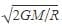Where M is the mass of the planet and R is the radius of the planet.
Thus we can see that v does not depend upon the mass of the object.

NEET Previous Year Questions: Gravitation - 1 - Question 3

### The kinetic energy needed to project a body of mass m from the earth's surface (radius R) to infinity is   [AIEEE 2002]

Detailed Solution for NEET Previous Year Questions: Gravitation - 1 - Question 3

If any object through with escaping velocity then the object will never come back at the ground, So we can assume it will reach at infinite distance.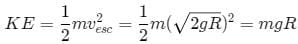NEET Previous Year Questions: Gravitation - 1 - Question 4

Energy required to move a body of mass m from an orbit of radius 2R to 3R is

[AIEEE 2002]

NEET Previous Year Questions: Gravitation - 1 - Question 5

The escape velocity for a body projected vertically upwards from the surface of earth is 11 km/s. If the body is projected at an angle of 45° with the vertical, the escape velocity will be

[AIEEE 2003]

Detailed Solution for NEET Previous Year Questions: Gravitation - 1 - Question 5

The escape velocity of a body does not depend on the angle of projection from earth. It is 11 km/s.
Hence C is correct.

NEET Previous Year Questions: Gravitation - 1 - Question 6

Two spherical bodies of mass M and 5M and radii R and 2R respectively are released in free space with initial separation between their centres equal to 12R. If they attract each other due to gravitational force only. then the distance covered by the smaller body just before collision is

[AIEEE 2003]

Detailed Solution for NEET Previous Year Questions: Gravitation - 1 - Question 6

Let the spheres collide after time t1 when the smaller sphere covered distance x1 and bigger sphere covered distance x2.
The gravitational force acting between two spheres depends on the distance which is a variable quantity.
The gravitational force,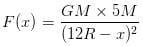Acceleration of smaller body,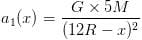Acceleration of bigger body,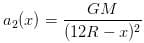From equation of motion,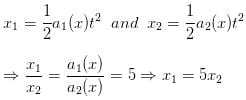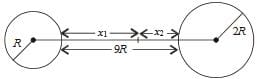We know that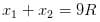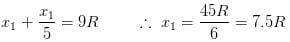Therefore the two spheres collide when the smaller sphere covered the distance of  7.5 R

NEET Previous Year Questions: Gravitation - 1 - Question 7

The time period of a satellite of earth is 5 h. If the separation between the earth and the satellite is increased to 4 times the previous value, the new time period will become

[AIEEE 2003]

Detailed Solution for NEET Previous Year Questions: Gravitation - 1 - Question 7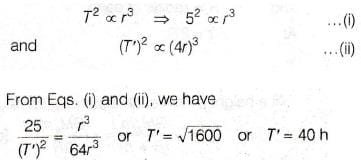NEET Previous Year Questions: Gravitation - 1 - Question 8

Suppose the gravitational forces varies inversely as the nth power of distance. Then the time period of a planet in circular orbit of radius R around the sun will be proportional to

[AIEEE 2004]

NEET Previous Year Questions: Gravitation - 1 - Question 9

If g is the acceleration due to gravity on the earth's surface, the gain in the potential energy of an object of mass m raised from the surface of the earth to a height equal to the radius R of the earth, is

[AIEEE 2004]

Detailed Solution for NEET Previous Year Questions: Gravitation - 1 - Question 9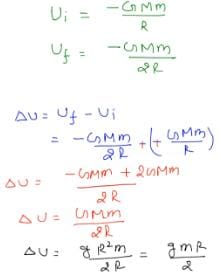NEET Previous Year Questions: Gravitation - 1 - Question 10

The time period of an earth satellite in circular orbit is independent of

[AIEEE 2004]

Detailed Solution for NEET Previous Year Questions: Gravitation - 1 - Question 10

T = 2π a/v
v = velocity of satellite
It is independent of mass of satellite

NEET Previous Year Questions: Gravitation - 1 - Question 11

A satellite of mass m revolves around the earth of radius R at a height x from its surface. If g is the acceleration due to gravity on the surface of the earth, the orbital speed of the satellite is

[AIEEE 2004]

Detailed Solution for NEET Previous Year Questions: Gravitation - 1 - Question 11

½ mv2 = GMm/2r
r = R + x
g = GM/R2
v = [gR2/R+x]2

NEET Previous Year Questions: Gravitation - 1 - Question 12

A particle of mass 10 g is kept on the surface of a uniform sphere of mass 100 kg and radius 10 cm. Find the work to be done against the gravitational force between them, to take the particle far away from the sphere, (you may take G = 6.67 × 10-11 Nm2/kg-2)

[AIEEE 2005]

Detailed Solution for NEET Previous Year Questions: Gravitation - 1 - Question 12

ΔW = Workdone = vf − ui
= 0 − [− GMmR]
ΔW = 6.67 × 10−11× 100 / 0.1 x 10/1000 = 6.67×10−10J

NEET Previous Year Questions: Gravitation - 1 - Question 13

The change in the value of g at a height h above the surface of the earth is the same as at a depth d below the surface of earth. When both and h are much smaller than the radius of earth, then which one of the following is correct ?

[AIEEE 2005]

NEET Previous Year Questions: Gravitation - 1 - Question 14

Average density of the earth

[AIEEE 2005]

NEET Previous Year Questions: Gravitation - 1 - Question 15

If gE and gM are the accelerations due to gravity on the surfaces of the earth and the moon respectively and if Millikan's oil drop experiment could be performed on the two surfaces, one will find the ratio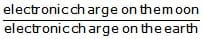to be

[AIEEE 2007]

Detailed Solution for NEET Previous Year Questions: Gravitation - 1 - Question 15

Since electronic charge (1.6 × 10–19C) is a universal constant. It does not depend on g. Therefore, electronic charge on the moon = electronic charge on the earth
Or
(electronic charge on the moon)/ (electronic charge on the earth) = 1

NEET Previous Year Questions: Gravitation - 1 - Question 16

Statement I : For a mass M kept at the centre of a cube of side a, the flux of gravitational field passing through its sides is 4p GM.

and

Statement II : If the direction of a field due to apoint source is radial and its dependence on the distance r from the source is given as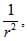its flux through a closed surface depends only on the strength of the source enclosed by the surface and not on the size or shape of the surface.

[AIEEE 2008]

Detailed Solution for NEET Previous Year Questions: Gravitation - 1 - Question 16

Gravity flux:
g × area = 4 π GM
Same as electric flux gravitation flux depends only on the strength of mass hot on shape as in electric flux it depend on the amount of charge not on shape or size of surface.

NEET Previous Year Questions: Gravitation - 1 - Question 17

A planet in a distant solar system is 10 times more massive than the earth and its radius is 10 times smaller. Given that the escape velocity from the earth is 11kms-1, the escape velocity from the surface of the planet would be

[AIEEE 2008]

Detailed Solution for NEET Previous Year Questions: Gravitation - 1 - Question 17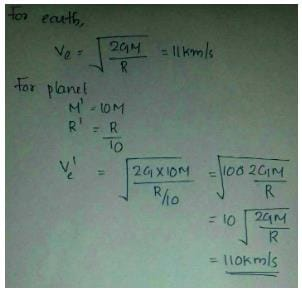NEET Previous Year Questions: Gravitation - 1 - Question 18

The height at which the acceleration due to gravity becomes g/9 (where g = the acceleration due to gravity on the surface of the earth) in terms of R, the radius of the earth is

[AIEEE 2009]

Detailed Solution for NEET Previous Year Questions: Gravitation - 1 - Question 18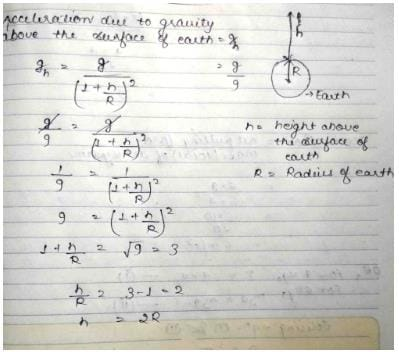NEET Previous Year Questions: Gravitation - 1 - Question 19

Two bodies of masses m and 4 m are placed at a distance r. The gravitational potential at a point on the line joining them where the gravitational field is zero, is

[AIEEE 2011]

Detailed Solution for NEET Previous Year Questions: Gravitation - 1 - Question 19

Let the point be the position when the gravitational field is zero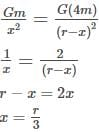the point P is at a distance r/3 from mass m and 2r/3 from mass 4m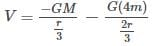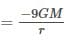NEET Previous Year Questions: Gravitation - 1 - Question 20

Two particles of equal mass m go around a circle of radius R under action of their mutual gravitational attraction. The speed of each particle with respect to their centre of mass is

[AIEEE 2011]

Detailed Solution for NEET Previous Year Questions: Gravitation - 1 - Question 20

Both their masses move in a circular path at exactly opposite diametrically ends with their centripetal forces being the common force of gravitation between them.
I.e. mv2/r = G.m.m/ 4r2
We get v =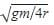NEET Previous Year Questions: Gravitation - 1 - Question 21

The mass of a spaceship is 1000kg. It is to be launched from the earth's surface out into free space. The value of g and R (radius of earth) are 10 m/s2 and 6400 Km respectively. The required energy for this work will be

[AIEEE 2012]

NEET Previous Year Questions: Gravitation - 1 - Question 22

What is the minimum energy required to launch a statellite of mass m from the surface of a planet of mass M and radius R in a circular orbit at an altitude of 2R?

[JEE MAIN 2013]

## Physics Class 11

127 videos|464 docs|210 tests
 Use Code STAYHOME200 and get INR 200 additional OFF Use Coupon Code
Information about NEET Previous Year Questions: Gravitation - 1 Page
In this test you can find the Exam questions for NEET Previous Year Questions: Gravitation - 1 solved & explained in the simplest way possible. Besides giving Questions and answers for NEET Previous Year Questions: Gravitation - 1, EduRev gives you an ample number of Online tests for practice

## Physics Class 11

127 videos|464 docs|210 tests

### How to Prepare for NEET

Read our guide to prepare for NEET which is created by Toppers & the best Teachers